# The bridge

A vehicle weighing 5,800 kg passes 41 km/h on an arched bridge with a radius of curvature of 62 m. What force is pushing the car onto the bridge as it passes through the center? What is the maximum speed it can cross over the center of the bridge so that it does not fly off the road?

F =  44764.139 N
v2 =  24.6621 m/s

### Step-by-step explanation: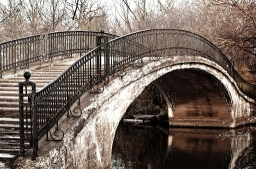Did you find an error or inaccuracy? Feel free to write us. Thank you!Tips to related online calculators
Do you have a linear equation or system of equations and looking for its solution? Or do you have a quadratic equation?
Do you want to convert mass units?
Do you want to convert velocity (speed) units?

#### You need to know the following knowledge to solve this word math problem:

We encourage you to watch this tutorial video on this math problem:

## Related math problems and questions:

• Car crashOn the road, with a maximum permitted speed of 60 km/h, there was a car crash. From the length of the vehicle's braking distance, which was 40 m, the police investigated whether the driver did not exceed that speed. What is the conclusion of the police, a
• The carThe car weight 1280 kg, increased its speed from 7.3 m/s to 63 km/h on a track of 37.2 m. What force did the car engine have to exert?
• Skid friction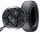Find the smallest coefficient of skid friction between the car tires and the road so that the car can drive at a 200 m radius at 108 km/h and does not skid.
• Dynamometer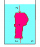What is the volume of a body that stretches a dynamometer in air, on which it is suspended by a force of 2.5 N, and if it is immersed in alcohol with a density of 800 kg/m ^ 3, does it tension the dynamometer with a force of 1.3 N?
• CarAt what horizontal distance reaches the car weight m = 753 kg speed v = 74 km/h when the car engine develops a tensile force F = 3061 N. (Neglect resistance of the environment.)
• Angled cyclist turnThe cyclist passes through a curve with a radius of 20 m at 25 km/h. How much angle does it have to bend from the vertical inward to the turn?
• Deceleration of carThe car has a speed of 60 km/h and after 100 m journey speed of 40 km/h. What is the deceleration of a car if we assume that its movement is constantly slowed down?
• Two boys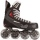Two boys weighing 60kg and 90kg move on roller skates and are attracted to each other by a rope. One boy exerts a force of 15N. What force does the other boy exert? What accelerations will the boys gain? Do not consider friction or air resistance.
• Positional energyWhat velocity in km/h must a body weighing 60 kg have for its kinetic energy to be the same as its positional energy at the height 50 m?
• The swing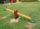To swing the two girls. Aneta weight 45 kg and Simon 35 kg weight. How far should sit Simon from the middle of swing so it is balanced, if we know that Aneta is sitting at distance 1,5m? How far are girls sitting apart?
• Two trainsThrough the bridge, long l = 240m, the train passes through the constant speed at time t1 = 21s. A train running along the traffic lights at the edge of the bridge passes the same speed at t2 = 9s. a) What speed v did the train go? b) How long did it take
• Propeller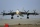The aircraft propeller rotates at an angular speed of 200 rad/s. A) What is the speed at the tip of the propeller if its distance from the axis of rotation is 1.5 m? B) What path does the aircraft travel during one revolution of the propeller at a speed o
• CarThe driver of a car is to get to 608 km distant city. From atlas found that 162 km will have to pass through the cities at average speed 48 km/h. Remainder of the journey pass outside the cities at average speed 116 km/h. Calculate how many hours it will
• A stone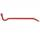There is a stone weighing 60 kg on the billet. The distance from the support point to the stone is 20cm. The length of the billet is 1m. Determine the force exerted by the hand at the end of the billet.
• Against each other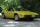From two points A, B distant 23 km at the same time started two cars against each other at speeds 41 km/h and 65 km/h. How long does cars meet and what distance passes each of them?
• 2 cyclists and carOne cyclist rides at a constant speed over a bridge. It is 100 meters long. When he is 40 meters behind him, he meets an oncoming cyclist who is riding at the same speed. The car travels along the bridge in the same direction as the first cyclist at a spe
• Speed of car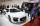The car went to a city that was 240 km away. If his speed increased by 8 km/h, it would reach the finish one hour earlier. Determine its original speed.